# Keynote Wheel of Persuasion - Bart Schutz of Online Dialogue on how to persuade system 1 and system 2 - with A/B-test examples

Oct. 23, 2014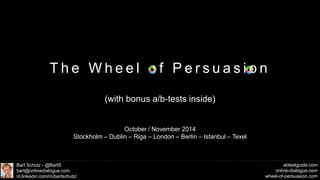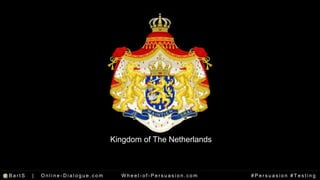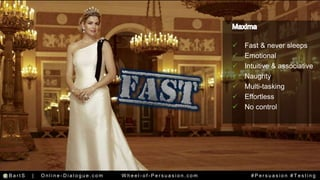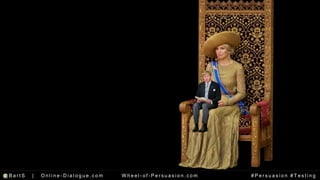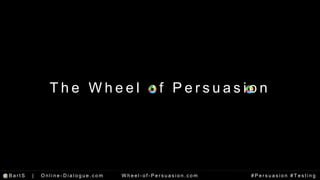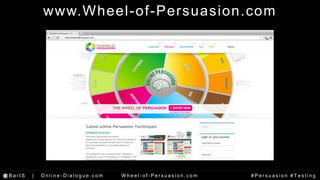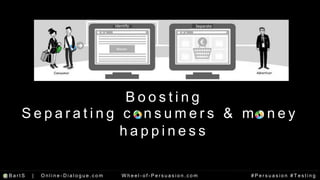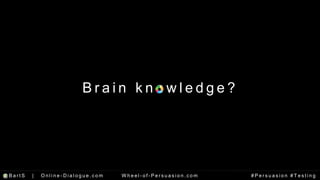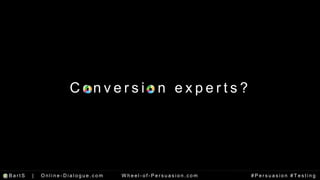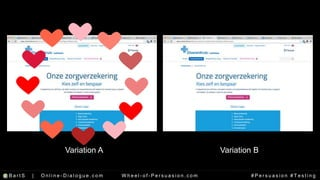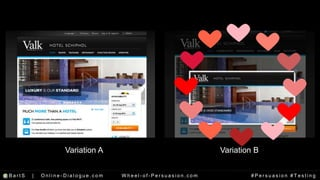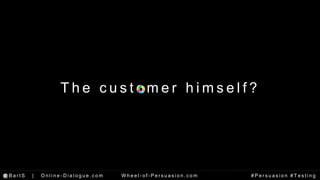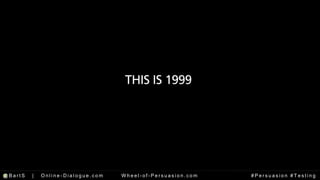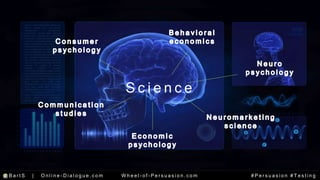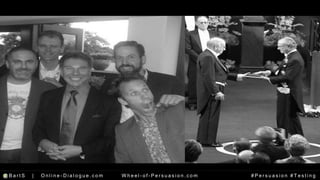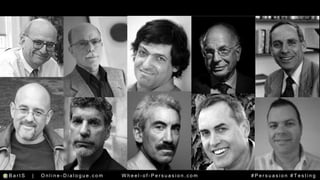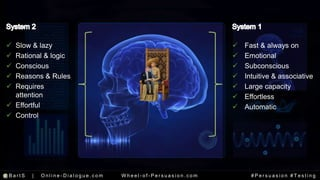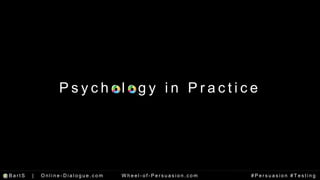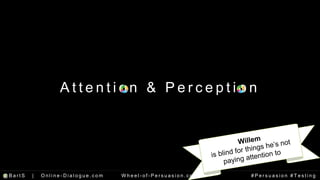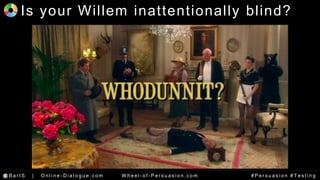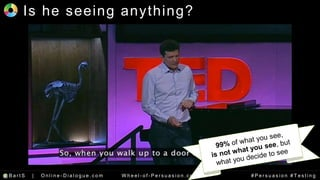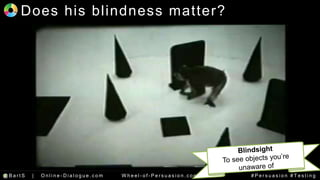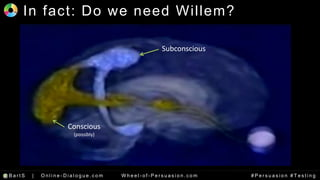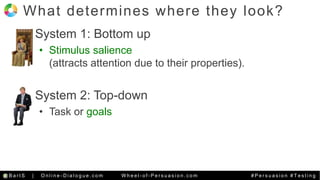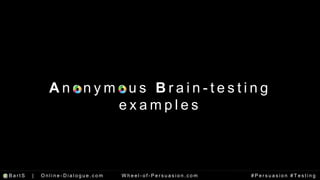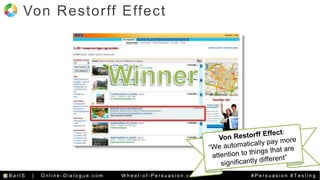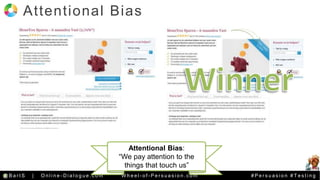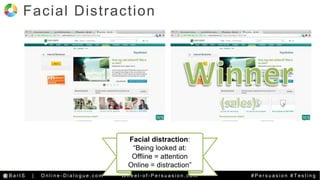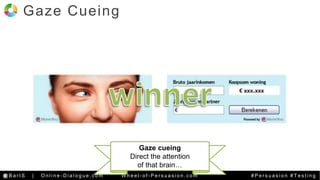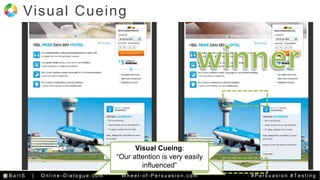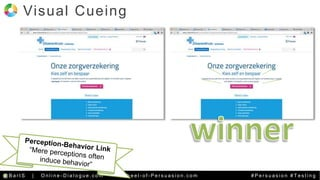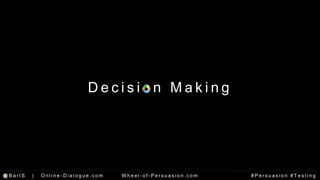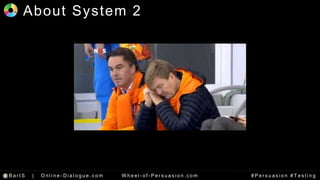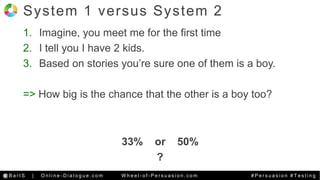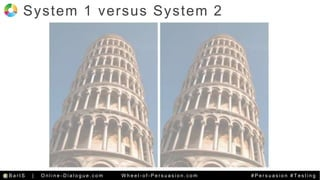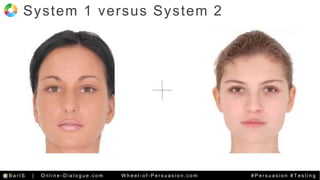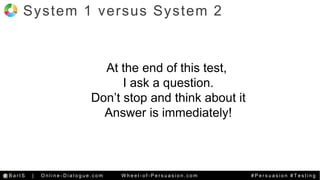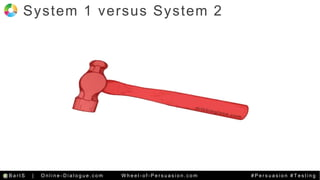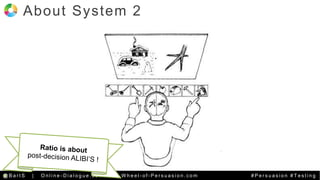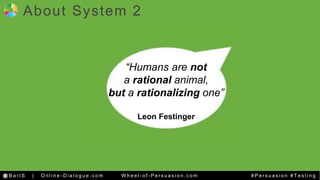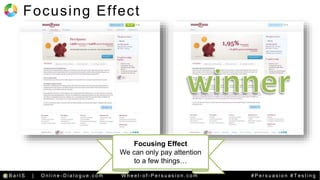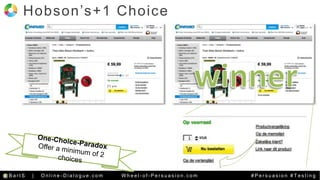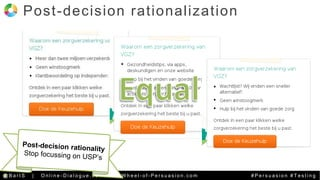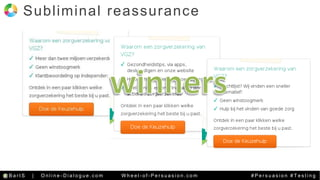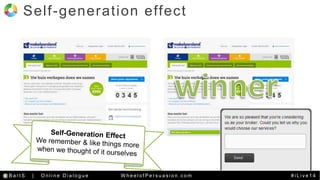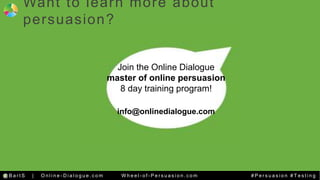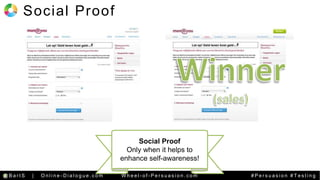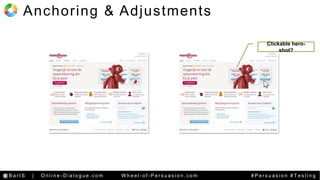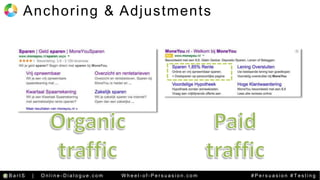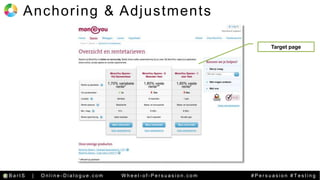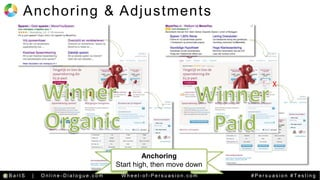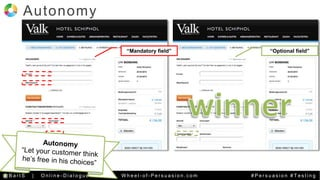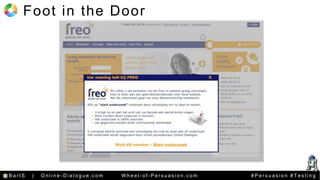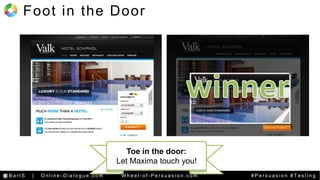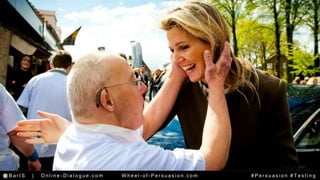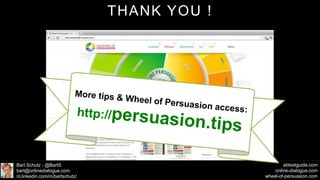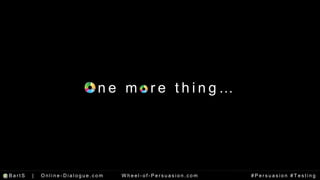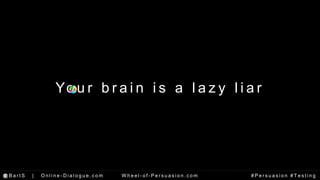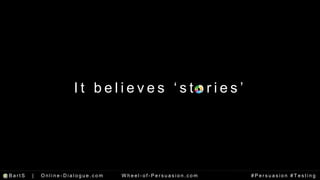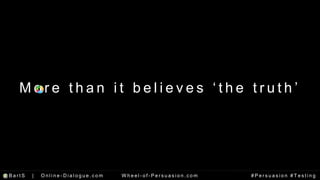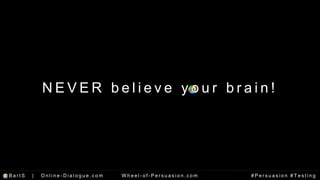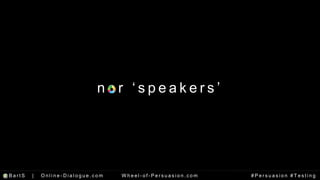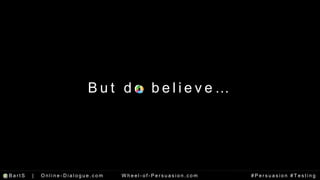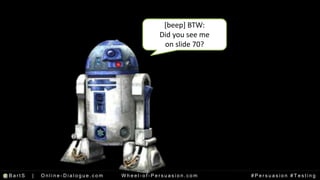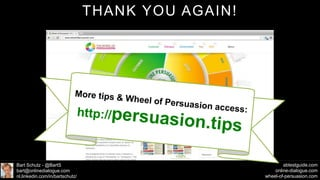1 of 94

### Keynote Wheel of Persuasion - Bart Schutz of Online Dialogue on how to persuade system 1 and system 2 - with A/B-test examples

• 1. T h e Wh e e l o f P e r s u a s i o n Bart Schutz - @BartS bart@onlinedialogue.com nl.linkedin.com/in/bartschutz/ abtestguide.com online-dialogue.com wheel-of-persuasion.com (with bonus a/b-tests inside) October / November 2014 Stockholm – Dublin – Riga – London – Berlin – Istanbul – Texel
• 2. Kingdom of The Netherlands @B a r t S | On l i n e - Di a l o g u e . c om Wh e e l - o f - P e r s u a s i o n . c om # P e r s u a s i o n # T e s t i n g
• 3. @B a r t S | On l i n e - Di a l o g u e . c om Wh e e l - o f - P e r s u a s i o n . c om # P e r s u a s i o n # T e s t i n g
• 4. @B a r t S | On l i n e - Di a l o g u e . c om Wh e e l - o f - P e r s u a s i o n . c om # P e r s u a s i o n # T e s t i n g
• 5. @B a r t S | On l i n e - Di a l o g u e . c om Wh e e l - o f - P e r s u a s i o n . c om # P e r s u a s i o n # T e s t i n g
• 6.  Slow & lazy  Rational  Reason & logic  Rules  Requires attention  Effortful  Controls Maxima @B a r t S | On l i n e - Di a l o g u e . c om Wh e e l - o f - P e r s u a s i o n . c om # P e r s u a s i o n # T e s t i n g
• 7. @B a r t S | On l i n e - Di a l o g u e . c om Wh e e l - o f - P e r s u a s i o n . c om # P e r s u a s i o n # T e s t i n g
• 8. @B a r t S | On l i n e - Di a l o g u e . c om Wh e e l - o f - P e r s u a s i o n . c om # P e r s u a s i o n # T e s t i n g
• 9. Filmpje Maxima met lijst kenmerken  Fast & never sleeps  Emotional  Intuitive & associative  Naughty  Multi-tasking  Effortless  No control @B a r t S | On l i n e - Di a l o g u e . c om Wh e e l - o f - P e r s u a s i o n . c om # P e r s u a s i o n # T e s t i n g
• 10. @B a r t S | On l i n e - Di a l o g u e . c om Wh e e l - o f - P e r s u a s i o n . c om # P e r s u a s i o n # T e s t i n g
• 11. T h e Wh e e l o f P e r s u a s i o n @B a r t S | On l i n e - Di a l o g u e . c om Wh e e l - o f - P e r s u a s i o n . c om # P e r s u a s i o n # T e s t i n g
• 12. ht tp: / /abtestguide.com/calc @B a r t S | On l i n e - Di a l o g u e . c om Wh e e l - o f - P e r s u a s i o n . c om # P e r s u a s i o n # T e s t i n g
• 13. Psychologist Psychologist Psychologist Psychologist Psychologist Psychologist Psychologist Psychologist Psychologist Psychologist Psychologist Psychologist Psychologist Psychologist Psychologist Psychologist Psychologist Psychologist @BartS Consumer psychologist @B a r t S | On l i n e Di a l o g u e Wh e e l o f P e r s u a s i o n . c om # i L i v e 1 4
• 14. www.Wheel -of-Persuasion.com @B a r t S | On l i n e - Di a l o g u e . c om Wh e e l - o f - P e r s u a s i o n . c om # P e r s u a s i o n # T e s t i n g
• 15. Identify Separate B o o s t i n g S e p a r a t i n g c o n s ume r s & mo n e y h a p p i n e s s @B a r t S | On l i n e - Di a l o g u e . c om Wh e e l - o f - P e r s u a s i o n . c om # P e r s u a s i o n # T e s t i n g
• 16. @B a r t S | On l i n e - Di a l o g u e . c om Wh e e l - o f - P e r s u a s i o n . c om # P e r s u a s i o n # T e s t i n g
• 17. B r a i n k n o w l e d g e ? @B a r t S | On l i n e - Di a l o g u e . c om Wh e e l - o f - P e r s u a s i o n . c om # P e r s u a s i o n # T e s t i n g
• 18. C o n v e r s i o n e x p e r t s ? @B a r t S | On l i n e - Di a l o g u e . c om Wh e e l - o f - P e r s u a s i o n . c om # P e r s u a s i o n # T e s t i n g
• 19. Variation A Variation B @B a r t S | On l i n e - Di a l o g u e . c om Wh e e l - o f - P e r s u a s i o n . c om # P e r s u a s i o n # T e s t i n g
• 20. Variation A Variation B @B a r t S | On l i n e - Di a l o g u e . c om Wh e e l - o f - P e r s u a s i o n . c om # P e r s u a s i o n # T e s t i n g
• 21. @B a r t S | On l i n e - Di a l o g u e . c om Wh e e l - o f - P e r s u a s i o n . c om # P e r s u a s i o n # T e s t i n g
• 22. Wilma Julia @B a r t S | On l i n e Di a l o g u e Wh e e l o f P e r s u a s i o n . c om # i L i v e 1 4
• 23. B r a i n k n o w l e d g e ? @B a r t S | On l i n e - Di a l o g u e . c om Wh e e l - o f - P e r s u a s i o n . c om # P e r s u a s i o n # T e s t i n g
• 24. T h e c u s t ome r h ims e l f ? @B a r t S | On l i n e - Di a l o g u e . c om Wh e e l - o f - P e r s u a s i o n . c om # P e r s u a s i o n # T e s t i n g
• 25. @B a r t S | On l i n e - Di a l o g u e . c om Wh e e l - o f - P e r s u a s i o n . c om # P e r s u a s i o n # T e s t i n g
• 26. @B a r t S | On l i n e - Di a l o g u e . c om Wh e e l - o f - P e r s u a s i o n . c om # P e r s u a s i o n # T e s t i n g
• 27. B r a i n k n o w l e d g e ? @B a r t S | On l i n e - Di a l o g u e . c om Wh e e l - o f - P e r s u a s i o n . c om # P e r s u a s i o n # T e s t i n g
• 28. S c i e n c e @B a r t S | On l i n e - Di a l o g u e . c om Wh e e l - o f - P e r s u a s i o n . c om # P e r s u a s i o n # T e s t i n g
• 29. @B a r t S | On l i n e - Di a l o g u e . c om Wh e e l - o f - P e r s u a s i o n . c om # P e r s u a s i o n # T e s t i n g
• 30. @B a r t S | On l i n e - Di a l o g u e . c om Wh e e l - o f - P e r s u a s i o n . c om # P e r s u a s i o n # T e s t i n g
• 31.  Slow & lazy  Rational & logic  Conscious  Reasons & Rules  Requires attention  Effortful  Control  Fast & always on  Emotional  Subconscious  Intuitive & associative  Large capacity  Effortless  Automatic @B a r t S | On l i n e - Di a l o g u e . c om Wh e e l - o f - P e r s u a s i o n . c om # P e r s u a s i o n # T e s t i n g
• 32. P s y c h o l o g y i n P r a c t i c e @B a r t S | On l i n e - Di a l o g u e . c om Wh e e l - o f - P e r s u a s i o n . c om # P e r s u a s i o n # T e s t i n g
• 33. A t t e n t i o n & P e r c e p t i o n @B a r t S | On l i n e - Di a l o g u e . c om Wh e e l - o f - P e r s u a s i o n . c om # P e r s u a s i o n # T e s t i n g
• 34. Is your Wi l lem inat tent ional ly bl ind? @B a r t S | On l i n e - Di a l o g u e . c om Wh e e l - o f - P e r s u a s i o n . c om # P e r s u a s i o n # T e s t i n g
• 35. Is he seeing anything? @B a r t S | On l i n e - Di a l o g u e . c om Wh e e l - o f - P e r s u a s i o n . c om # P e r s u a s i o n # T e s t i n g
• 36. Does his bl indness mat ter? @B a r t S | On l i n e - Di a l o g u e . c om Wh e e l - o f - P e r s u a s i o n . c om # P e r s u a s i o n # T e s t i n g
• 37. In fact : Do we need Wi l lem? • Conscious versus subconscious processing Subconscious Conscious (possibly) @B a r t S | On l i n e - Di a l o g u e . c om Wh e e l - o f - P e r s u a s i o n . c om # P e r s u a s i o n # T e s t i n g
• 38. What determines where they look? System 1: Bottom up • Stimulus salience (attracts attention due to their properties). System 2: Top-down • Task or goals @B a r t S | On l i n e - Di a l o g u e . c om Wh e e l - o f - P e r s u a s i o n . c om # P e r s u a s i o n # T e s t i n g
• 39. A n o n ymo u s B r a i n - t e s t i n g e x amp l e s @B a r t S | On l i n e - Di a l o g u e . c om Wh e e l - o f - P e r s u a s i o n . c om # P e r s u a s i o n # T e s t i n g
• 40. Von Restor ff Effect @B a r t S | On l i n e - Di a l o g u e . c om Wh e e l - o f - P e r s u a s i o n . c om # P e r s u a s i o n # T e s t i n g
• 41. At tent ional Bias Attentional Bias: “We pay attention to the things that touch us” @B a r t S | On l i n e - Di a l o g u e . c om Wh e e l - o f - P e r s u a s i o n . c om # P e r s u a s i o n # T e s t i n g
• 42. Facial Dist ract ion Facial distraction: “Being looked at: Offline = attention Online = distraction” @B a r t S | On l i n e - Di a l o g u e . c om Wh e e l - o f - P e r s u a s i o n . c om # P e r s u a s i o n # T e s t i n g
• 43. Gaze Cueing Gaze cueing Direct the attention of that brain… @B a r t S | On l i n e - Di a l o g u e . c om Wh e e l - o f - P e r s u a s i o n . c om # P e r s u a s i o n # T e s t i n g
• 44. Visual Cueing Visual Cueing: “Our attention is very easily influenced” @B a r t S | On l i n e - Di a l o g u e . c om Wh e e l - o f - P e r s u a s i o n . c om # P e r s u a s i o n # T e s t i n g
• 45. Visual Cueing @B a r t S | On l i n e - Di a l o g u e . c om Wh e e l - o f - P e r s u a s i o n . c om # P e r s u a s i o n # T e s t i n g
• 46. ht tp: / / test ing.agency @B a r t S | On l i n e - Di a l o g u e . c om Wh e e l - o f - P e r s u a s i o n . c om # P e r s u a s i o n # T e s t i n g
• 47. D e c i s i o n Ma k i n g @B a r t S | On l i n e - Di a l o g u e . c om Wh e e l - o f - P e r s u a s i o n . c om # P e r s u a s i o n # T e s t i n g
• 48. About System 2 @B a r t S | On l i n e - Di a l o g u e . c om Wh e e l - o f - P e r s u a s i o n . c om # P e r s u a s i o n # T e s t i n g
• 49. System 1 versus System 2 1. Imagine, you meet me for the first time 2. I tell you I have 2 kids. 3. Based on stories you’re sure one of them is a boy. => How big is the chance that the other is a boy too? 33% or 50% ? @B a r t S | On l i n e - Di a l o g u e . c om Wh e e l - o f - P e r s u a s i o n . c om # P e r s u a s i o n # T e s t i n g
• 50. System 1 versus System 2 @B a r t S | On l i n e - Di a l o g u e . c om Wh e e l - o f - P e r s u a s i o n . c om # P e r s u a s i o n # T e s t i n g
• 51. System 1 versus System 2 @B a r t S | On l i n e - Di a l o g u e . c om Wh e e l - o f - P e r s u a s i o n . c om # P e r s u a s i o n # T e s t i n g
• 52. System 1 versus System 2 At the end of this test, I ask a question. Don’t stop and think about it Answer is immediately! @B a r t S | On l i n e - Di a l o g u e . c om Wh e e l - o f - P e r s u a s i o n . c om # P e r s u a s i o n # T e s t i n g
• 53. System 1 versus System 2 @B a r t S | On l i n e - Di a l o g u e . c om Wh e e l - o f - P e r s u a s i o n . c om # P e r s u a s i o n # T e s t i n g
• 54. About System 2 @B a r t S | On l i n e - Di a l o g u e . c om Wh e e l - o f - P e r s u a s i o n . c om # P e r s u a s i o n # T e s t i n g
• 55. About System 2  Bart Schutz  dr. Dirk Franssens “Humans are not a rational animal, but a rationalizing one” Leon Festinger @B a r t S | On l i n e - Di a l o g u e . c om Wh e e l - o f - P e r s u a s i o n . c om # P e r s u a s i o n # T e s t i n g
• 56. A n o n ymo u s B r a i n - t e s t i n g e x amp l e s @B a r t S | On l i n e - Di a l o g u e . c om Wh e e l - o f - P e r s u a s i o n . c om # P e r s u a s i o n # T e s t i n g
• 57. Focusing Effect Focusing Effect We can only pay attention to a few things… @B a r t S | On l i n e - Di a l o g u e . c om Wh e e l - o f - P e r s u a s i o n . c om # P e r s u a s i o n # T e s t i n g
• 58. H o b s o n ’s + 1 C h o i c e @B a r t S | On l i n e - Di a l o g u e . c om Wh e e l - o f - P e r s u a s i o n . c om # P e r s u a s i o n # T e s t i n g
• 59. H o b s o n ’s + 1 C h o i c e @B a r t S | On l i n e - Di a l o g u e . c om Wh e e l - o f - P e r s u a s i o n . c om # P e r s u a s i o n # T e s t i n g
• 60. Post -decision rat ional izat ion @B a r t S | On l i n e - Di a l o g u e . c om Wh e e l - o f - P e r s u a s i o n . c om # P e r s u a s i o n # T e s t i n g
• 61. Subl iminal reassurance @B a r t S | On l i n e - Di a l o g u e . c om Wh e e l - o f - P e r s u a s i o n . c om # P e r s u a s i o n # T e s t i n g
• 62. Sel f -generat ion effect @B a r t S | On l i n e - Di a l o g u e . c om Wh e e l - o f - P e r s u a s i o n . c om # P e r s u a s i o n # T e s t i n g Wh e e l o f P e r s u a s i o n . c om # i L i v e 1 4
• 63. Want to learn more about persuasion?  Bart Schutz  dr. Dirk Franssens Join the Online Dialogue master of online persuasion 8 day training program! info@onlinedialogue.com @B a r t S | On l i n e - Di a l o g u e . c om Wh e e l - o f - P e r s u a s i o n . c om # P e r s u a s i o n # T e s t i n g
• 64. Social Proof Social Proof Only when it helps to enhance self-awareness! @B a r t S | On l i n e - Di a l o g u e . c om Wh e e l - o f - P e r s u a s i o n . c om # P e r s u a s i o n # T e s t i n g
• 65. Anchor ing & Adjustments 1.80% 1.85% 1.80% 1.85% Clickable hero-shot? @B a r t S | On l i n e - Di a l o g u e . c om Wh e e l - o f - P e r s u a s i o n . c om # P e r s u a s i o n # T e s t i n g
• 66. Anchor ing & Adjustments @B a r t S | On l i n e - Di a l o g u e . c om Wh e e l - o f - P e r s u a s i o n . c om # P e r s u a s i o n # T e s t i n g
• 67. Anchor ing & Adjustments Target page @B a r t S | On l i n e - Di a l o g u e . c om Wh e e l - o f - P e r s u a s i o n . c om # P e r s u a s i o n # T e s t i n g
• 68. Anchor ing & Adjustments 1.80% 1.85% 1.80% 1.85% Anchoring Start high, then move down X @B a r t S | On l i n e - Di a l o g u e . c om Wh e e l - o f - P e r s u a s i o n . c om # P e r s u a s i o n # T e s t i n g
• 69. Autonomy “Mandatory field” “Optional field” @B a r t S | On l i n e - Di a l o g u e . c om Wh e e l - o f - P e r s u a s i o n . c om # P e r s u a s i o n # T e s t i n g
• 70. Foot in the Door @B a r t S | On l i n e - Di a l o g u e . c om Wh e e l - o f - P e r s u a s i o n . c om # P e r s u a s i o n # T e s t i n g
• 71. Foot in the Door Toe in the door: Let Maxima touch you! @B a r t S | On l i n e - Di a l o g u e . c om Wh e e l - o f - P e r s u a s i o n . c om # P e r s u a s i o n # T e s t i n g
• 72. @B a r t S | On l i n e - Di a l o g u e . c om Wh e e l - o f - P e r s u a s i o n . c om # P e r s u a s i o n # T e s t i n g
• 73. @B a r t S | On l i n e - Di a l o g u e . c om Wh e e l - o f - P e r s u a s i o n . c om # P e r s u a s i o n # T e s t i n g
• 74. @B a r t S | On l i n e - Di a l o g u e . c om Wh e e l - o f - P e r s u a s i o n . c om # P e r s u a s i o n # T e s t i n g
• 75. @B a r t S | On l i n e - Di a l o g u e . c om Wh e e l - o f - P e r s u a s i o n . c om # P e r s u a s i o n # T e s t i n g
• 76. @B a r t S | On l i n e - Di a l o g u e . c om Wh e e l - o f - P e r s u a s i o n . c om # P e r s u a s i o n # T e s t i n g
• 77. THANK YOU ! Bart Schutz - @BartS bart@onlinedialogue.com nl.linkedin.com/in/bartschutz/ abtestguide.com online-dialogue.com wheel-of-persuasion.com
• 78. O n e m o r e t h i n g … @B a r t S | On l i n e - Di a l o g u e . c om Wh e e l - o f - P e r s u a s i o n . c om # P e r s u a s i o n # T e s t i n g
• 79. Yo u r b r a i n i s a l a z y l i a r @B a r t S | On l i n e - Di a l o g u e . c om Wh e e l - o f - P e r s u a s i o n . c om # P e r s u a s i o n # T e s t i n g
• 80. I t b e l i e v e s ‘ s t o r i e s ’ @B a r t S | On l i n e - Di a l o g u e . c om Wh e e l - o f - P e r s u a s i o n . c om # P e r s u a s i o n # T e s t i n g
• 81. M o r e t h a n i t b e l i e v e s ‘ t h e t r u t h ’ @B a r t S | On l i n e - Di a l o g u e . c om Wh e e l - o f - P e r s u a s i o n . c om # P e r s u a s i o n # T e s t i n g
• 82. S o … @B a r t S | On l i n e - Di a l o g u e . c om Wh e e l - o f - P e r s u a s i o n . c om # P e r s u a s i o n # T e s t i n g
• 83. N E V E R b e l i e v e y o u r b r a i n ! @B a r t S | On l i n e - Di a l o g u e . c om Wh e e l - o f - P e r s u a s i o n . c om # P e r s u a s i o n # T e s t i n g
• 84. n o r ‘ s p e a k e r s ’ @B a r t S | On l i n e - Di a l o g u e . c om Wh e e l - o f - P e r s u a s i o n . c om # P e r s u a s i o n # T e s t i n g
• 85. B u t d o b e l i e v e … @B a r t S | On l i n e - Di a l o g u e . c om Wh e e l - o f - P e r s u a s i o n . c om # P e r s u a s i o n # T e s t i n g
• 86. y o u r D ATA ! ! ! @B a r t S | On l i n e - Di a l o g u e . c om Wh e e l - o f - P e r s u a s i o n . c om # P e r s u a s i o n # T e s t i n g
• 87. @B a r t S | On l i n e - Di a l o g u e . c om Wh e e l - o f - P e r s u a s i o n . c om Wh e e l o f P e r s u a s i o n . c om # P e r s u a s i o n ##Ti Leisvtei n1 g4
• 88. @B a r t S | On l i n e - Di a l o g u e . c om Wh e e l - o f - P e r s u a s i o n . c om Wh e e l o f P e r s u a s i o n . c om # P e r s u a s i o n ##Ti Leisvtei n1 g4
• 89. CRO? => GROW your business! Analyze & Experiment Digital Data Persuasion Psychology Be aware: 70% of A/B-tests are wrong! http://abtestguide.com @B a r t S | On l i n e - Di a l o g u e . c om Wh e e l - o f - P e r s u a s i o n . c om # P e r s u a s i o n # T e s t i n g
• 90. ht tp: / /abtestguide.com/calc @B a r t S | On l i n e - Di a l o g u e . c om Wh e e l - o f - P e r s u a s i o n . c om # P e r s u a s i o n # T e s t i n g
• 91. ht tp: / / test ing.agency @B a r t S | On l i n e - Di a l o g u e . c om Wh e e l - o f - P e r s u a s i o n . c om # P e r s u a s i o n # T e s t i n g
• 92. Want to learn more about persuasion?  Bart Schutz  dr. Dirk Franssens Join the Online Dialogue master of online persuasion 8 day training program! info@onlinedialogue.com @B a r t S | On l i n e - Di a l o g u e . c om Wh e e l - o f - P e r s u a s i o n . c om # P e r s u a s i o n # T e s t i n g
• 93. [beep] BTW: Did you see me on slide 70? @B a r t S | On l i n e - Di a l o g u e . c om Wh e e l - o f - P e r s u a s i o n . c om # P e r s u a s i o n # T e s t i n g
• 94. THANK YOU AGAIN! Bart Schutz - @BartS bart@onlinedialogue.com nl.linkedin.com/in/bartschutz/ abtestguide.com online-dialogue.com wheel-of-persuasion.com

### Editor's Notes

1. He married Maxima
2. Slide 71